# 15. Volume¶

The Volume tab contains commands for manipulating 3D and 4D datasets. This includes cropping, signal scaling, smoothing, application of edge-enhancing filters and so on. Also included in this tab are tools for conversion of numeric data type between integer and real and changing data dimensions.

## 15.1. Window Center/Width setting¶

These commands automatically adjust the grayscale window center and width to the optimal settings for selected conditions. See also View > Grayscale Window for details of grayscale window center and width settings.

This operation is defined for integer and real volumes only. The optimal window center/width setting is defined as follows. First, all non-void (non-transparent) voxels are sorted into a list by their signal intensity values. Next, a fixed percentage of all voxels (0.5%) is removed from the top and the bottom of the list. For the remaining voxels, the range between the lowest and highest signal values is taken to be the optimal window width and the midpoint of this range as the optimal window center.

### 15.1.1. Optimal for all slices and timepoints¶

Automatically adjusts grayscale window center and width to the values optimal for viewing of all slices and timepoints for a dynamic dataset.

### 15.1.2. Optimal for current timepoint¶

Automatically adjusts window center and width to the values optimal for viewing of the current timepoint (time frame). May be convenient for viewing dynamic series in which image intensity varies in a wide range, such as dynamic contrast-enhanced MRI or CT images showing high signal enhancement in blood vessels during the passage of the contrast bolus with.

### 15.1.3. Optimal for current ROI¶

Automatically adjusts window center and width to the values optimal for viewing of the active, visible ROI layer. If the document contains two or more ROI layers, but the active layer is not an ROI, shows an error message (Ambiguous Layer Configuration).

## 15.2. Advance to (timepoint)¶

Commands in this group help to quickly navigate to an automatically selected frame of a dynamic dataset.

### 15.2.1. Timepoint of Maximum Info over ROI¶

Selects and displays the dynamic frame with the maximum information within a raster ROI. Requires a 4D (dynamic) dataset and a single raster ROI. If the document contains two or more ROI layers, shows an error message (Ambiguous layer configuration). If the document contains no visible ROI, displays the dynamic frame with the maximum information for the entire image.

In FireVoxel, by default, images (volumes) are compressed to save RAM. Each slice of the volume is compressed separately using three-voxel predictive coding combined with the Huffman entropy encoder. The compressed size of the entire volume is thus the sum of the sizes of individual slices.

The size of the compressed volume is an indicator of how much information is contained in the volume. The less uniform the signal within the volume, the more information it contains and the larger the volume’s compressed size. A uniform volume (a volume containing the same signal values throughout) has nearly zero compressed size.

This command advances to the timepoint where a 3D volume has the largest compressed size. In dynamic contrast-enhanced studies, which consist of a series of 3D volumes, the maximum information usually corresponds to the timepoint of the maximum contrast enhancement.

Users may select non-compressed internal representation of the volumes via File > User Interface Options > RAM Compress Integer volumes (uncheck). Without compression, all slices and timepoints have the same amount of information, and operations based on maximum information are not meaningful.

### 15.2.2. Timepoint of Vector ROI peak¶

Selects and displays the time frame showing maximum image intensity within the active vector ROI (VROI). If the VROI is not present, or multiple VROIs are present, but none is selected, FireVoxel will show a corresponding error message (if no VROIs: At least one seed is expected; if multiple VROIs, none selected: With several seeds present, a single one should be selected). The seed VROI may be 2D, 3D, or 4D.

## 15.3. Crop¶

Selects a user-specified subset of the original dataset. Creates a new document window (named [original]_cropped) displaying the selected subset.

### 15.3.1. Crop with 4D Vector ROI – Active Layer only¶

Requires a document with an active layer and an active vector ROI (VROI).

Selects a part of the active layer enclosed by the VROI. Creates a new document window (named [original]_cropped) displaying the selected subset of the active layer. The VROI is not copied to the cropped document.

The active layer may contain an image (3D or 4D), raster ROI (3D or 4D), or a color map. Even if the active layer is invisible, it will be cropped and displayed as visible in the new (cropped) document window.

The VROI may be 2D, 3D, or 4D. A 2D or 3D VROI will crop a single slice, volume, or dynamic frame on which it is defined. Similarly, a 4D VROI will crop only those dynamic frames on which it is defined.

To adjust the dynamic frames, double left-click the VROI to open VROI Properties panel and enter the dynamic index interval. See Vector for details.

If multiple VROIs are present in the document, but none is selected, the command shows an error message (More than one Vector ROI is present and none selected).

If the document contains multiple layers, they are not included into the cropped document. To crop multiple layers, use Crop with 4D Vector ROI — All Layers.

### 15.3.2. Crop with 4D Vector ROI – All Layers¶

Requires a document with one or more layers and an active vector ROI (VROI).

Selects a part of all layers in the document enclosed by the VROI. Creates a new document window (named [original]_cropped) displaying the selected subset of the original document. The VROI is not copied to the cropped document.

The original document may contain images (3D or 4D), raster ROIs (3D or 4D), or color maps. Both visible and invisible layers will be cropped, but invisible layers will remain invisible in the new (cropped) document window.

The VROI may be 2D, 3D, or 4D. A 2D or 3D VROI will crop a single slice, volume, or dynamic frame on which it is defined. Similarly, a 4D VROI will crop only those dynamic frames on which it is defined.

To adjust the dynamic frames, double left-click the VROI to open VROI Properties panel and enter the dynamic index interval. See Vector for details.

If multiple VROIs are present in the document, but none is selected, the command shows an error message (More than one Vector ROI is present and none selected).

### 15.3.3. Crop current timepoint¶

Requires a dynamic (4D) image. Selects a single frame (3D volume) at the current value of dynamic variable (such as time, b-value, flip angle, inversion time, Dixon contrast, etc.) Creates a new document window (named [original_image]_cropped) showing the selected (cropped) volume.

The dynamic variable and dynamic index are shown in the status bar at the bottom left corner of the software window (Fig. 30.2).

If the original document contains other visible layers (including ROI layers), they are copied into the cropped document. The layers that are invisible in the original document are not included into the cropped document. Vector ROIs are not retained.

The original document remains unchanged.

### 15.3.4. Crop current slice¶

Requires a 3D or 4D image in the active document window. Selects the current slice (e.g., slice number P) from the original document. Creates a new document window (labeled [original]_slP) showing the selected slice.

If the original document contains a 3D image, the cropped image is a 2D slice. If the original document contains a 4D image, the cropped image is a 2D slice at all dynamic points of the original document. All raster ROI layers (both visible and invisible) and vector ROIs are replicated in the cropped document.

### 15.3.5. Extract specified dynamic points¶

Requires a 4D image. Opens a dialog prompting the user to enter the indices of the dynamic variable to be selected. These indices may be separated by commas (e.g., 1, 3, 9) to select individual dynamic frames, or by dashes (e.g., 1-10) to select a range of indices.

Creates a new document window (labeled [original]_cropped) with a 4D image that includes the 3D volumes with the specified dynamic variable indices.

For example, the user selects time points 1, 3, 9 from a time series, the cropped image will contain volumes at time points number 1, 3, and 9. If the user selects dynamic points 1-10, the cropped image will contain the first ten images of the dynamic series.

### 15.3.6. Remove specified dynamic points¶

Requires a 4D image. Opens dialog prompting the user to enter an index (or indices) of the dynamic variable of the 3D volumes that will be removed from the original 4D image.

Creates a new document window (labeled [original]_cropped) with a 4D image in which the specified dynamic frames are absent.

If the dynamic variable is time (with the time points from t1=0 s to tN), and the first K points are removed [t1, tK], the time points of the remaining images [tK+1, tN] are adjusted by subtracting tK+1 so that the time points of the cropped image are in the interval of [0 s, tN — tK+1].

If the original document contains invisible layers, they are not replicated in the cropped document. However, visible layers (images, ROIs, maps) are retained.

Requires a document with an active layer (visible or invisible). Opens dialog (Specify Pad size (mm)) to enter padding size in millimeters.

Creates a new document window (named [active_window]_pad) with padded image, in which zero intensity voxels added to each dimension of the original volume.

The dimensions of the padded image are (w+p, h+p, d+p), where w, h, and d are the width, height and depth of the original image (in mm), and p is the pad size. If the image is 4D, each frame of the original dynamic series is padded. The voxel size of the padded image is the same as the original voxel size. The matrix of the padded image is increased by the number of added padding voxels.

## 15.5. Noise¶

Returns the noise values estimated using different methods for the image in the active layer. Displays the results in FireVoxel image processing dialog. Use Ctrl+C, Ctrl+V to copy and paste these results elsewhere.

### 15.5.1. Effective Noise¶

Requires an integer image. Returns the value of effective noise.

This is effectively a 2D algorithm. The algorithm scans all slices within the volume and for each slice measures the average signal within a 2D window (10 mm x 10 mm). The window with the lowest average signal and assumed to contain air. The effective noise is then computed from the Rayleigh distribution: Mean_Signal = Noise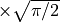.

### 15.5.2. Background Noise¶

Requires an integer image or a real image without VOID voxels. Returns the value of background noise.

The original volume is first converted to Isotropic Edge Voxels. Next, the 3D edges in the volume are detected using proprietary Texture Edge Detector algorithm. All voxels whose six-voxel neighborhood (in 3D) does NOT contain edge voxels are considered to be central voxels. For such central voxels, the algorithm computes the difference:

D = |Signal(Center) – AverageOfSixNeighbors(Center)|.

This difference is accumulated and its average value is calculated for the entire volume. The resulting average value is background noise.

### 15.5.3. Median Noise¶

Requires an integer or real image. Returns the value of median noise.

The volume is first smoothed with the 3D median filter with radius R = 1. The purpose of median smoothing is to remove the noise without disturbing the volume edges. The difference between the original and smoothed voxels is then computed over the entire volume. This difference is averaged over all voxels and is returned as median noise.

### 15.5.4. Local 2D Difference Noise¶

Requires an integer or real image. Returns the value of local difference noise.

Calculates the average differences between each voxel and four of its nearest horizontal and vertical neighbors (in 2D). Computes this difference for all voxels. Sorts differences by value, then takes the lower half of this sorted array (to presumably exclude edges) and calculates the average noise (local difference noise).

## 15.6. Smooth over (optional) ROI¶

Computes a smoothed image by convolving the original image with a normalized 3D spherical (if 3D smoothing is selected) or circular (if 2D is selected) kernel, or filter, with user-selected radius.

Creates a new layer with smoothed image.

Requires a 3D or 4D image, integer or real, in the active layer. For a 4D (dynamic) dataset, each dynamic frame is smoothed independently. May use an optional ROI to perform smoothing only within this ROI.

Once called, the command opens dialog to adjust the smoothing parameters (Volume Smooth parameters, Fig. 15.1).Fig. 15.1 Volume Smooth parameter dialog.

Parameters:

• Process 3D (checkbox) – Selects spherical (3D, if checked) or circular (2D, if unchecked) smoothing.

• Kernel Type – Smoothing kernel options include: Uniform (default), Gaussian, Radial, Raleigh, Median, Minimal SI, and Maximal SI.

• Radius (vox) – Filter radius (in voxels).

• Constrain by Mask (checkbox) – If checked, the visible ROI is used to perform smoothing only within this ROI. If the document contains two or more visible ROIs, this option is unavailable. To use it, uncheck visibility of all ROIs except one.

Example:

Consider a 2D uniform (kernel type: Uniform) smoothing using a filter with the radius of 2 voxels. FireVoxel convention is to define a single voxel as having the radius equal to 0. This means that the diameter of the circle of radius 2 voxels is 5 voxels (diameter = 2 x radius + 1). The “circular” and symmetric kernel with weights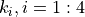, is shown below:

(15.1)In this example, the kernel has 13 non-zero weights. Since the kernel is uniform,. All smoothing kernels are normalized, meaning that the sum of 13 weights is 1.0. So in this example allweights are equal to.

The smoothing operation works as follows. The kernel is positioned so that the central element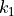is above a target voxel. The values of 25 image voxels located under this kernel are multiplied by the corresponding weightsand all products are summed. The resulting sum is the voxel value of the target voxel in the smoothed image. The kernel is then moved to the next voxel and the process is repeated until the entire smoothed image is computed.

For the uniform kernel, the weightsare all equal, and as a result, the smoothed voxel is the average of its 13 neighboring voxels. For the Gaussian kernel, the weightsare given by the values of a Gaussian function.

## 15.7. Smooth across Dynamic dimension¶

Computes a smoothed dynamic image. Requires a 4D image – integer, real, or 4D raster ROI.

Creates a new layer with the smoothed image.

Once called, the command opens dialog (Specify integer) to select Dynamic Smooth Radius, or the number of dynamic frames over which the intensity will be smoothed.

Smoothing is performed for each 3D coordinate (x,y,z) independently. For the dynamic coordinate value N and user-selected dynamic smooth radius Rad, the signal intensity values are averaged for all voxels within the dynamic coordinate interval [N-Rad, N+Rad]. Void voxels are excluded from averaging. If a voxel (x,y,z) at dynamic variable N is VOID, it remains VOID in the smoothed image.

## 15.8. Volume Edge-constrained Smooth¶

Computes a smoothed image within areas constrained by automatically determined edges.

Requires a 3D or 4D image, integer or real, in the active layer. For a 4D (dynamic) image, each dynamic frame is smoothed independently. Creates a new, integer layer with the smoothed image.

Once called, the command opens dialog to adjust the smoothing parameters (Edge-Constrained Smooth, Fig. 15.2):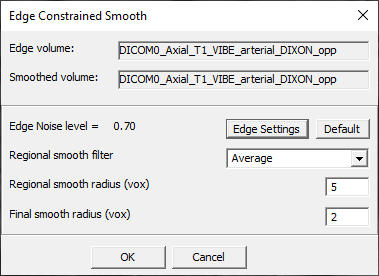Fig. 15.2 Volume Edge-Constrained Smooth dialog.

Parameters:

• Edge volume – Folder location of the edge volume.

• Smoothed volume – Folder location of the smoothed volume.

• Edge noise level [value] – Noise level measured…

• Edge Settings – Access to a secondary dialog for adjusting the Edge Settings (3D Edge Detector). Default – button resets Edge Settings to defaults.

• Regional smooth filter – Kernel type of the regional filter. Options include: Average (default), Median, Gaussian.

• Regional smooth radius (vox) – Radius of the smoothing aperture (in voxels).

• Final smooth radius (vox) – Radius (in voxels)…

## 15.9. Inter-Volume Edge-Constrained Smooth¶

Requires a 4D (dynamic) image (?).

If the image in the active layer is incompatible, shows an error message (No suitable imag`present or enabled). Creates a new layer with the smoothed image (real-valued, grayscale).

Once called, the command opens dialog (Edge-Constrained Smooth, same as Fig. 15.2) to adjust the operation parameters.

## 15.10. Non-local Means Denoising¶

Computes a denoised image using a non-local means (NLM) filter. Requires a 3D or 4D image, integer or real, in the active layer. If the image is 4D (dynamic), applies denoising to each dynamic frame independently.

Creates a new layer with denoised image.

Once called, the command opens dialog (Non-local Means Denoising, Fig. 15.3) to enter the parameters of the NLM filter.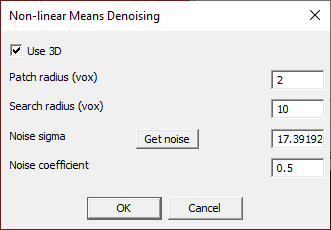Fig. 15.3 Non-local Means Denoising Dialog.

This procedure is based on the most popular non-local means (NLM) denoising algorithm [Buades2005]. The non-local means filter takes the mean of all voxels in the image weighted by the similarity of these voxels’ neighborhood to the neighborhood of the target voxel. FireVoxel extends the original 2D algorithm to 3D and makes it fully parallel to gain speed.

Buades A, Coll B, Morel J-M. A non-local algorithm for image denoising. Computer Vision and Pattern Recognition, 2005. Vol. 2. pp. 60–65. doi:10.1109/CVPR.2005.38.

Parameters:

• Use 3D – If checked, applies the 3D patch and searches over the entire volume.

• Patch radius (vox) – The size (in voxels) of the spherical patch contained within the specified (real) radius. Typically, the radius is in the [1,5] interval. Note: Processing time grows roughly as radius^3 (radius cubed), and higher radius values must be done judiciously.

• Search radius (vox) – The size of the rectangular area where search and accumulation of the similarity score is performed. Theoretically, this size should be equal to the entire volume. However, since the execution time grows roughly as the radius^3 (radius cubed), typically search radius=10 is used. Higher or lower values may be used depending on the user’s hardware.

• Noise sigma – A measure of the noise level. Controlled by the user to achieve how strong denoising is.

• Get Noise (button) – Calculates the Local Difference noise to give the user an anchor value for operation.

• Noise coefficient – Enables the choice between the two similar formulas in the NLM implementation paper. Note: Build 373 – This parameter is currently not used, but will be implemented in the future versions to allow access to the second formula.

## 15.11. Texture Edge Detector with optional Constraint ROI¶

Requires a 3D or 4D image, integer or real. May also use an optional constraint ROI. Creates a new layer with the edge map (real-valued, rainbow colormap by default).

When the command is selected:

• If the document contains a visible ROI layer, a dialog pops up: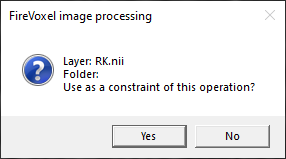• If Yes is selected, then processing will be done only within this ROI. If No is selected, then the entire image will be processed. If the document contains two or more ROI layers, an error message is shown (Ambiguous layer configuration).

• If the image is 4D, a dialog appears (Process all timepoints?):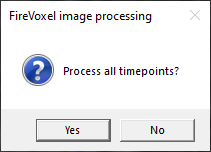• If Yes is selected, each dynamic frame will be processed independently and the result will also be a 4D image. If No is selected, only the current frame will be processed, and the result is 3D.

• Next, the main dialog appears (3D Edge Detector, Fig. 15.4) to adjust the parameters of edge detector. Processing starts once the user clicks OK.

## 15.12. 3D Edge Detector¶

The 3D Edge Detector dialog (Fig. 15.4) is used by several commands, including Volume > Volume Edge-constrained smooth and Inter-Volume Edge-constrained smooth, among others.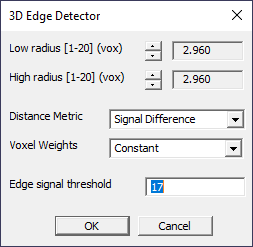Fig. 15.4 3D Edge Detector Dialog.

Parameters:

• Low radius\High radius [1-20] (vox) – Lowest and highest size of features calculated (in voxels). Default: 2.960 voxels.

• Distance Metric – Calculation type for histogram comparison. The options include Signal Difference or Histogram EMD (Earth Mover Distance).

• Voxel Weights – Choice of voxel weights to account for the voxel distance to the aperture center. The options include Constant, Gaussian, Radial, and Rayleigh.

• Edge signal threshold – Signal intensity threshold.

## 15.13. Single Scale Texture Gradient¶

Calculates texture gradient for the user-selected single level of features (characteristic size). This function uses an optional ROI (in which case the analysis is performed only within this ROI) and also optionally processes all timepoints in a 4D image. When the command is selected:

• If a visible ROI is present, a question pops up: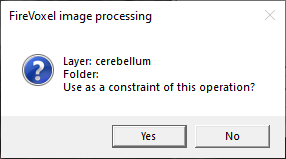• If Yes is selected, the gradient map is calculated only within the selected ROI. If No is selected, the gradient map is computed for the entire image.

• Next, if the original image is a 4D image, a dialog box pops up:• If Yes is selected, the operation is applied to all timepoints (each dynamic frame is analyzed independently) and the resulting gradient map is also 4D. If No is selected, the operation is applied only to the current timepoint and the resulting gradient map is 3D.

• Next, the main dialog appears (Single Scale Texture Gradient, Fig. 15.5) and processing starts once the user clicks OK.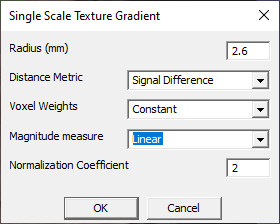Fig. 15.5 Single Scale Texture Gradient dialog.

Parameters:

• Radius (mm) – Level (characteristic size, in millimeters) of the image features calculated.

• Distance Metric – Calculation type for histogram comparison. The options include Signal Difference or Histogram EMD (Earth Mover Distance).

• Voxel Weights – Choice of voxel weights to account for the voxel distance to the aperture center. The options include Constant, Gaussian, Radial, and Rayleigh.

• Magnitude measure – Filter response value is converted to the output value based on 3 different schemes: Linear, Logarithm, Normalized.

• Normalization CoefficientCoefficient used in Magnitude Measure=Normalize.

## 15.14. Multiscale Texture Gradient¶

Calculates texture gradient for the range of feature levels and for each voxel selects the maximum magnitude of the gradient. During segmentation, different regions may be better separated at different feature levels, and therefore an operation probing a range of feature levels may be able to separate these regions. This function can also use an optional ROI and optionally process all timepoints. When the command is selected:

• If a visible ROI is present, a question pops up:• If Yes is selected, the resulting gradient map will be calculated only within the selected ROI. If No is selected, the gradient is calculated over the entire image.

• Next, if the original image is a 4D image, a dialog box pops up:• If Yes is selected, the operation is applied to all timepoints (each dynamic frame is analyzed independently) and the resulting gradient map is also 4D. If No is selected, then the operation is applied only to the current timepoint (or dynamic frame) and the resulting gradient map is 3D.

• Next, the main dialog box appears (Multiscale Texture Gradient, Fig. 15.6) and processing starts once the user clicks OK.Fig. 15.6 Multiscale Texture Gradient dialog.

Parameters:

• Low radius\High radius (vox) – Specifies the range of features calculated (in voxels). Note the difference from the Single Scale Gradient radius, which is in mm. Default: 2.6 voxels.

• Distance Metric – Calculation type is Signal Difference or Histogram EMD (Earth Mover Distance).

• Voxel Weights – Choice of voxels weights to account for voxel distance to the aperture center.

• Magnitude Measure – Filter response value is converted to the output value based on 3 different schemes: Linear, Logarithm, Normalized.

## 15.15. Multiscale Texture Gradient with 4D Aggregate¶

This function is similar to the regular multiscale texture gradient, but works only for 4D Volumes. During a dynamic exam, the best separation between various pairs of regions may be achieved at different timepoints. To take advantage of these optimal separations, the function calculates the Multiscale Texture Gradient for each timepoint separately. Then 4D Aggregate operation is performed, when for each voxel the maximum gradient magnitude is selected across all timepoints and assigned to the resulting map. Note that the result of this operation is always a 3D gradient map. When the command is selected:

• If a visible ROI is present, a question pops up:

• If Yes is selected, the gradient map is calculated only within the ROI.

• Next, the main dialog box appears (the same interface as for Multiscale Texture Gradient, Fig. 15.6) and processing starts once the user clicks OK.

Parameters:

• Low radius\High radius (vox) – Specify the lowest and highest size of features calculated (in voxels). Note the difference to the Single Scale Gradient radius, which is in mm. Default: 2.6 voxels.

• Distance Metric – Calculation type includes Signal Difference or Histogram EMD (Earth Mover Distance).

• Voxel Weights – Choice of voxels weights to account for voxel distance to the aperture center. The options include Constant, Gaussian, Radial, and Rayleigh.

• Magnitude Measure – Filter response value is converted to the output value based on 3 different schemes: Linear, Logarithm, Normalized.

## 15.16. Depth to Time¶

Requires a 4D image with dimensions W x H x D x N, where W, H, and D are width, height and depth, respectively, and N is the number of dynamic frames. Creates a new document window named [active_window]_DtoT displaying the current dynamic frame as W x H x 1 x D image. To scroll through slices, the user may use Right and Left arrow keys on the keyboard.

If applied again to the W x H x 1 x D image, creates a new document window displaying only the current slice (W x H x 1 x 1).

## 15.17. Time to Depth¶

Requires a single slice across multiple dynamic points, W x H x 1 x N (for other dimensions, shows an error message: Volume should contain a single z-slice.) Creates a new document window named [active_window]_TtoD with reformatted image with dimensions W x H x N x 1. If used again on this new image, shows an error message: 4D volume required.

## 15.18. Copy voxels from current timepoint to target timepoint¶

Opens dialog (Specify Integer) to enter the target dynamic point index where the current dynamic frame will be copied. Copies, voxel-by-voxel, the current 3D dynamic frame onto the specified dynamic frame.

## 15.19. Adaptive Threshold¶

Build 373+: See ROI > Local Otsu Threshold to ROI.

## 15.20. Convert 2D Mosaic to Volume¶

Requires a mosaic image (2D). Mosaic is a Siemens format for storing and displaying a 3D image as a 2D, square array of slices shown as tiles from top left, row by row, to bottom right.

If the original 3D image has voxel dimensions of W x H x D (in the column, row, and slice directions, respectively), the dimensions of the mosaic image are (p x W) x (p x H), where p is an integer (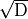rounded up to the next integer). Thus, the mosaic is the smallest square array that accommodates all slices of the original image.

Opens dialog (Specify Integer) to enter the number of images in the mosaic. The default number of images is obtained from the private DICOM tag 0019,100A (Number of images in mosaic).

Transforms the mosaic into a regular 3D image with W x H x D dimensions. Creates a new document window displaying the transformed image.

Other relevant tags are: Acquisition Matrix (0018,1310) (frequency rows\frequency columns\phase rows\phase columns), Rows (0028,0010), Columns (0028,0011).

In a regular 3D image, the Columns tag is usually equal to the frequency columns value in the Acquisition Matrix. For example, for a 3D image, the image dimensions tags may be as follows:
0018,0093 Percent sampling 80
0018,1310 Acquisition Matrix 0\320\256\0
0028,0010 Rows 320
0028,0011 Columns 320

In a mosaic, the Acquisition Matrix tags indicate the dimensions of the individual images, whereas the Rows and Columns tags contain the dimensions of the larger mosaic image, equal to the multiples (p) of the dimensions of the individual images. For example, for a 5 x 5 mosaic:
0018,1310 Acquisition Matrix 0\80\62\0
0028,0010 Rows 400
0028,0011 Samping 310

Note that these tags indicate the dimensions of the individual images and the minimum dimensions of the square table required to accommodate this 3D image, but not the total number of images.

## 15.21. Volume type conversion¶

These commands convert images into different data types. Converted images are placed in new, automatically created layers.

### 15.21.1. Real to integer¶

Requires a visible real-valued image (in the active layer or another layer). Opens dialog (Specify Integer) to enter the number of Resulting Bits for integer-valued image (15 by default). Creates a new layer in the active document window (named [original_layer]_integer) with the original layer converted to integer-valued image. The new layer is displayed with the same grayscale window width and level as the original layer.

If the active layer is not a real-valued image and there are no other visible real-valued layers, an error message is shown (Real valued volume is required). If the active layer is not a real-valued image, but there are two or more visible real-valued layers, the first visible layer (as listed in the Layer Control) is converted.

### 15.21.2. Integer to real¶

Requires integer-valued image in the active layer. Creates a new layer (named [original_layer]_real) containing the original layer converted to a real-valued image.

If the active layer is not an integer-valued image, an error message is shown (Signal intensity volume is required).

### 15.21.3. ROI to integer¶

Requires a visible ROI layer. Creates a new layer (named [original]_integer) with voxel values obtained from the voxel values of the original layer converted to integer signal intensity. The new layer is displayed as a rainbow colormap with the window width/level of 1/0.5 and opacity of 100%.

If the active layer is not an ROI layer, and there is no visible ROI layer in the document, the command shows an error message (Signal intensity volume is required).

## 15.22. Voxel Value Conversion¶

### 15.22.1. Equalize Histogram¶

Formerly Volume > Voxel Value Conversion > Flatten Histogram.

The algorithm has been revised to match the classic description of the Histogram Equalization algorithm.

Requires a 3D integer image in the active layer. Does not work for any other images. There are no parameters for this operation.

Creates a new layer in the same document window (named after as the original layer). The distribution of intensities of the resulting image may be examined using Layer Control > ROI Stats 3D.

### 15.22.2. Linear conversion over ROI¶

Attention

Linear conversion modifies voxel values of the original image. Use Undo/Redo toolbar features if dissatisfied with results.

Keywords: scale, rescale, multiply, divide, normalize, ratio

Applies linear transformation to the image intensity values. Requires an active image layer. May also be used with a visible raster ROI layer. If the document has a visible ROI, the transformation is applied only within this ROI. If a visible ROI is not present, or if there are two or more visible ROIs in the document, the conversion is applied to the entire image.

Opens dialog (Linear Conversion, Fig. 15.7) to specify the parameters of linear conversion:

S1 = C x S0 + B,

where C is the coefficient, B is the bias, and converted S1 is truncated within the user-specified signal interval [Low Limit, High Limit].

The operation replaces voxel intensities of the original image (S0) with the transformed values (S1) within the ROI (if a visible ROI is present) or within the entire image (if an ROI is not present). The S1 values that fall below the Low Limit or above the High Limit are replaced with the corresponding limit values.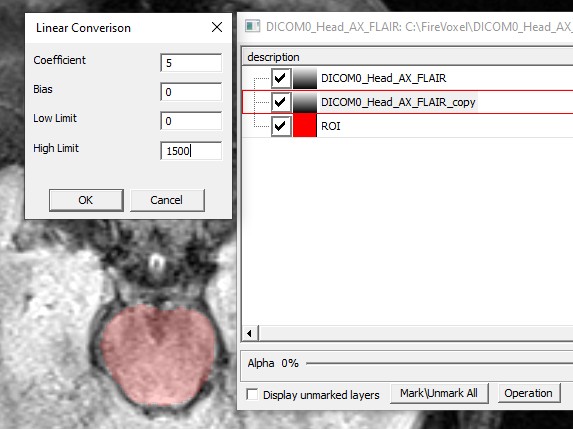Fig. 15.7 Voxel value linear conversion over ROI.

Example: Scale the image signal intensity within a selected area down by a factor of 7.

1. Open Layer Control.

2. Draw an ROI manually over the selected area or use segmentation tools such as EdgeWave Segmentation. If the area contains only a few voxels, use Paintbrush and enter Radius of 0 voxels in Paintbrush Properties to select individual voxels one by one. Make sure that the ROI layer is visible.

3. Activate the image layer in Layer Control by clicking on its name.

4. Select Volume > Voxel value conversion > Linear conversion over ROI. Enter Coefficient = 0.143 (= 1/7), Bias = 0, and appropriate Low and High Limit values (Fig. 15.7). Click OK.

5. The intensity values within the ROI will be replaced with the scaled values. If necessary, use Window and Level tool to adjust the grayscale window for optimal viewing of the transformed values.

6. If dissatisfied with the result, use Undo function to revert to the original state. Adjust the transformation parameters and repeat the operation with these new parameters.

### 15.22.3. Invert¶

Requires an image in the active document.

Attention

Invert modifies voxel values of the original image. Use Undo/Redo toolbar features if dissatisfied with results.

For real and integer volumes: Replaces the original voxel intensities S0 with symmetrical intensities about the middle of the interval [S0min, S0max]. The distributions of the original and inverted signal intensities can be examined using the histogram using Layer Control > ROI Stats 3D.

For ROI layers: Changes the value of each voxel to the inverted value (0<—>1): vnew = 1—v, where v and vnew are the old and the new (inverted) voxel values, respectively.

Note: If you wish to modify only the appearance of the image (rather than the image intensity values), use Layer Control > View Filter and check Invert Scale checkbox. This option inverts the display of grayscale (the order of gray values) without changing the voxel values in the volume.

### 15.22.4. Logarithm Conversion¶

Requires an image in the active layer.

Creates a new, real-valued layer in which voxel intensities S1 are a log function of the original intensities S0: S1= log(S0). By default, the new layer is displayed as a rainbow color map.

### 15.22.5. Scale to Interval¶

Requires an image (integer or real) in the active layer.

Modifies voxel values of the original image. The original voxel signal intensities S0 are replaced with transformed intensities S1. Opens dialog (Specify Signal Range) to enter [S1min, S1max], the minimum and maximum values of the transformed intensity.

The original voxel intensities in the active layer, S0, are replaced with transformed intensities S1(x,y,z) = S1min + (S0(x,y,z) – S0min) x (S1max – S1min)/(S0max – S0min).

### 15.22.6. Change Specified Signal to Transparent¶

Requires an image in the active layer.

Modifies voxel intensities of the original image. Opens dialog (Specify Make Specified Signal Transparent) to enter signal intensity value to be replaced with a transparent voxel. Replaces voxels with user-specified intensities with transparent voxels.

### 15.22.7. Change Transparent to Specified Signal¶

Requires an image in the active layer, presumably containing transparent voxels. Modifies voxel intensities of the original image.

Opens dialog (Specify New value for transparent signal) to enter the signal intensity value to be assigned to transparent voxels. Replaces transparent voxels with opaque voxels with this user-specified intensity value.

## 15.23. Insert Uniform Layer¶

Opens dialog (Specify Uniform signal value) to specify the signal intensity value.

Creates a new, real-valued layer with all voxels having this user-specified intensity. The new layer is shown as a colormap and is placed on top of all existing layers in the document.

## 15.24. Set DICOM tags¶

Opens dialog (Specify string) to enter MRI sequence parameters. Modality is not checked. The new values overwrite the original value of the corresponding DICOM field.

### 15.24.1. Set TR (Repetition time)¶

Enter TR in ms. The new value is written into DICOM field RepetitionTime (18,0080).

### 15.24.2. Set TE (Echo time)¶

Enter TE in ms. The new value is written into DICOM field EchoTime (18,0081).

### 15.24.3. Set FA (Flip angle)¶

Enter Flip Angle in degrees. The new value is written into DICOM field FlipAngle (18,1314).

## 15.25. Set Volume Orientation¶

Opens dialog (Specify string) to enter a sequence of four letters, specifying the orientation of the volume with respect to the screen directions, [Top, Bottom, Left, Right]. The letters should always form a 4-letter combination without spaces or punctuation marks: SIRL, APLR, etc. If the user enters implausible orientation, a warning will be displayed.

The allowed direction labels are: L — left, R — right, A — anterior, P — posterior, S — superior, I — inferior.

Example: If the volume orientation is set to LRAP

• top of the screen becomes L,

• bottom — R,

• left — A,

• right — P.

## 15.26. Change Patient Position and Orientation¶

Opens dialog (Specify Image Position/Orientation Patient, Fig. 15.8) to change the position and orientation of the patient.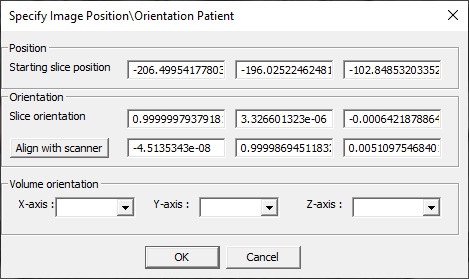Fig. 15.8 Specify Image PositionOrientation Patient panel.

The Position and Orientation coordinates are read from DICOM header fields ImagePositionPatient (20,0032) for starting slice and ImageOrientationPatient (20,0037).

Clicking Align with scanner changes the orientation so that for each slice the orientation axis is aligned with one of the scanner axes (1,0,0), (0,1,0), or (0,0,1).